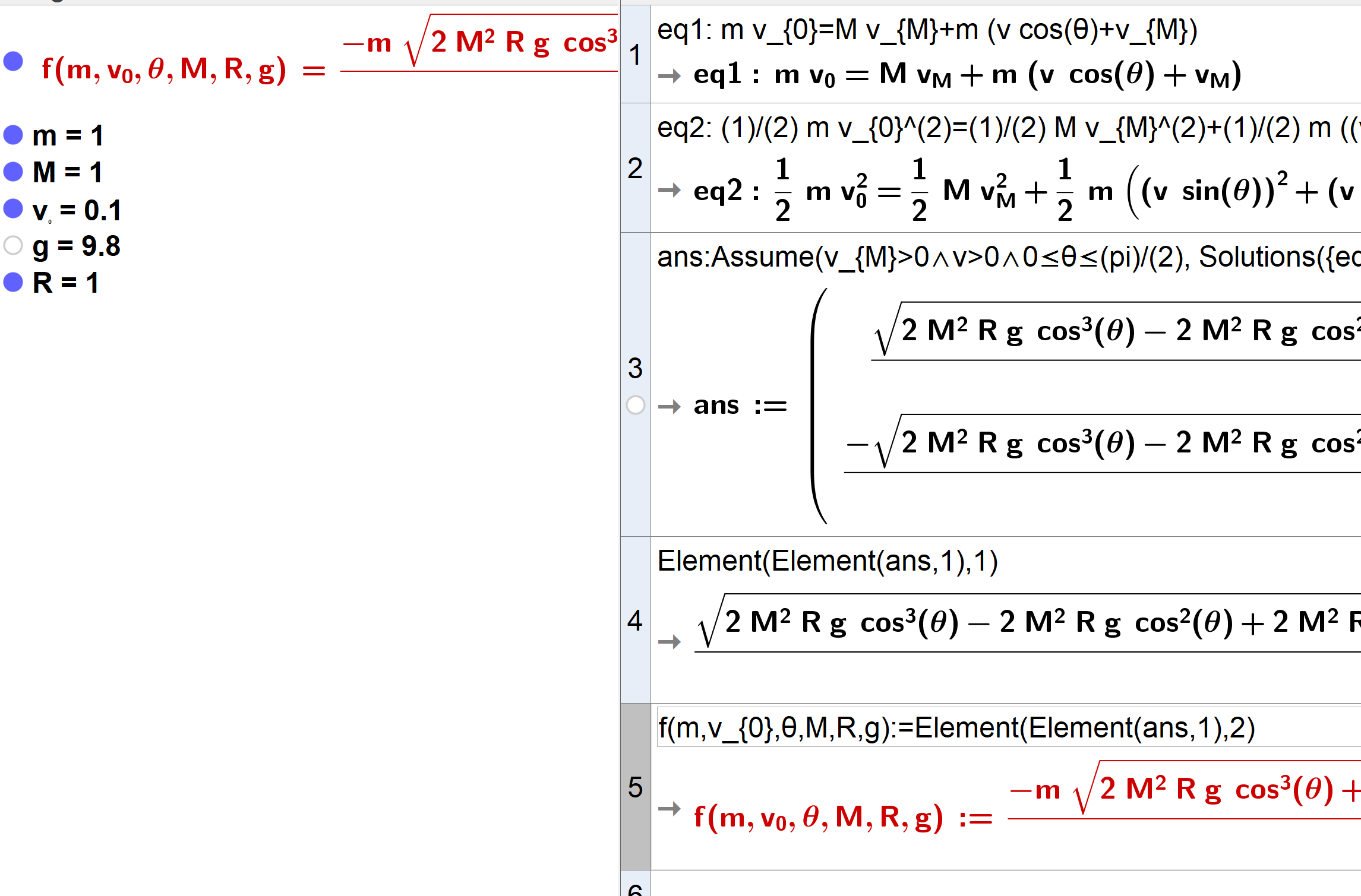# How to transfer long expressions from CAS to Algebra?

Nicholasfox shared this question 2 years ago

I solved a system of equations in CAS, and would like to continue using the solutions in Algebra. How to transfer long expressions from CAS to Algebra?Bug fixed in v6521

1) Use Solutions(), not Solve()

```ans:Assume(v_{M}>0∧v>0∧0≤θ≤(pi)/(2), Solutions({eq1,eq2},{v,v_{M}}))
```
2) Define it as a function like

`f(m,v_{0},θ,M,R,g):=Element(Element(ans,1),2)`1

Got it. Thank you very much.

A little problem, it works, but f2() displayed in Algebra area is different from f1().